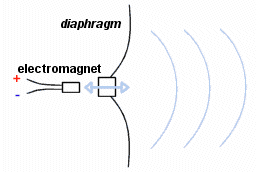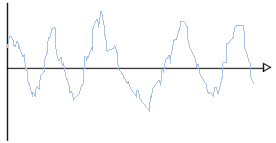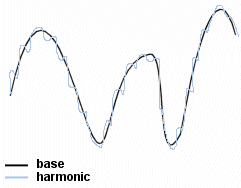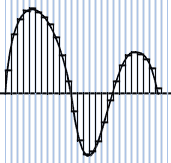# Digital Audio

## What is sound?

Sound is vibrations in the air; that is, a series of rising and falling pressures in the air, deviating from the average, which is represented by atmospheric pressure. To prove this, you could place something loud (like an alarm clock) inside a vacuum chamber, and notice that the initially noisy object no longer makes a sound if it isn't surrounded by air anymore.

The simplest way to create a sound is to make an object vibrate. In this manner, a violin makes a sound when the bow makes its strikes vibrate, and a piano sounds a note when a key is struck, because a hammer struck a string and made it vibrate.

Speakers are generally used to reproduce these sounds. They are a membrane connected to an electromagnet; as an electrical current travels in front of and behind the magnet very rapidly, it causes vibrations in the air in front of it, and that vibration is sound!This is how sound waves are produced; they can be represented in a diagram as changes in air pressure (or in the electricity level of the magnet) as a function of time. This can be depicted as:This sort of graphic depiction of sound is called amplitude modulation (modulation of the amplitude of a sound over time). A sonogram, on the other hand, depicts sound frequencies as a function of time. It should be noted that a sonogram shows fundamental frequency, on top of which higher frequencies, called harmonics, are superimposed.This is what allows us to distinguish between different sources of sound: low notes have low frequencies, while high notes have higher frequencies.

## Sound sampling

To play sound on a computer, it must be converted into a digital format, as this is the only kind of information computers can work with. A computer program intersperses small samples of the sound (which amount to differences in pressure) at specific intervals of time. This is called sampling or digitising sound. The period of time between two samples is called the sampling rate. As reproducing audio which sounds continuous to the ear requires samples at least once every few 100,000ths of a second, it is more practical to go by the number of samples per second, expressed in Hertz (Hz). Here are a few examples of common sampling rates, and what sound quality they correspond to:

Sampling rate Sound quality
44,100 Hz CD quality
22,000 Hz Radio quality
8,000 Hz Telephone quality

The sampling rate of an audio CD, for example, is not arbitrary. In fact, it follows from Shannon's theorem. Sampling frequency must be high enough to preserve the form of the signal. The Nyquist-Shannon theorem stipulates that the sampling rate must be equal to or greater than twice the maximum frequency contained in the signal. Our ears can hear sounds up to about 20,000 Hz. Therefore, for a satisfactory level of sound quality, the sampling rate must be at least on the order of 40,000 Hz. There are several standardized sampling rates in use:

• 32 kHz: for digital FM radio (band-limited to 15 kHz)
• 44.1 kHz: for professional audio and compact discs
• 48 kHz: for professional digital multitrack recording, and consumer recording equipment (like DAT or MiniDisc)

## Sound for computers

Each sample (corresponding to an interval of time) is associated with a value, which determines the air pressure value at that moment. Therefore, sound is not depicted as a continuous curve with deviations, but as a series of values for each interval of time:A computer works with bits, so the number of possible values that the sample could have must be determined. This is done by setting the number of bits on which the sample values are encoded.

• With 8-bit coding, there are 28 (= 256) possible values.
• With 16-bit coding, there are 216 (= 65536) possible values.

The second option clearly offers higher sound fidelity, but at the cost of using more computer memory.

Finally, stereo sound requires two channels, with sound recorded individually on each one. One channel is fed into the left speaker, while the other is broadcast from the right speaker.

In computer processing, a sound is therefore represented by several parameters:

• The sampling rate
• The number of bits in a sample
• The number of channels (one for mono, two for stereo, and four for quadrophonic sound)

## Memory required for storing a sound file

It is easy to calculate what size an uncompressed audio sequence will be. By knowing how many bits are used to code the sample, you know its size (as the sample size is the number of bits)

To find out the size of a channel, all you need to know is the sample rate, and thus the number of samples per second, and from that the amount of space taken up by one second of music. This comes to:
Sampling rate x Number of bits

Therefore, to find out how much space a sound extract several seconds long will take up, just multiply the preceding value by the number of seconds:
Sampling rate x Number of bits x Number of seconds

Finally, to determine the actual file size of the extract, the above figure should be multiplied by the number of channels (it will be twice as large for stereo as for mono).
The size, in bits, of a sound extract is therefore equal to:

Sampling rate x Number of bits x Number of seconds x Number of channels